Minima/ maxima (statement questions) - Geometry questions

Chapter 6 Class 12 Application of Derivatives
Concept wise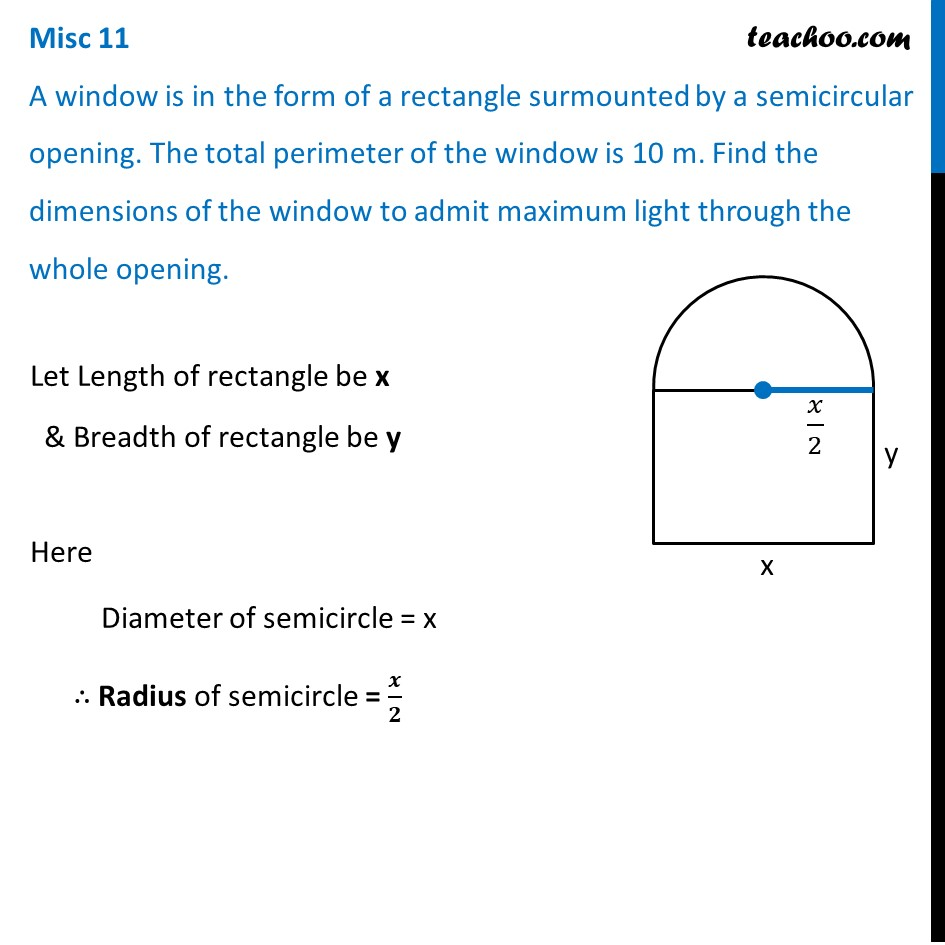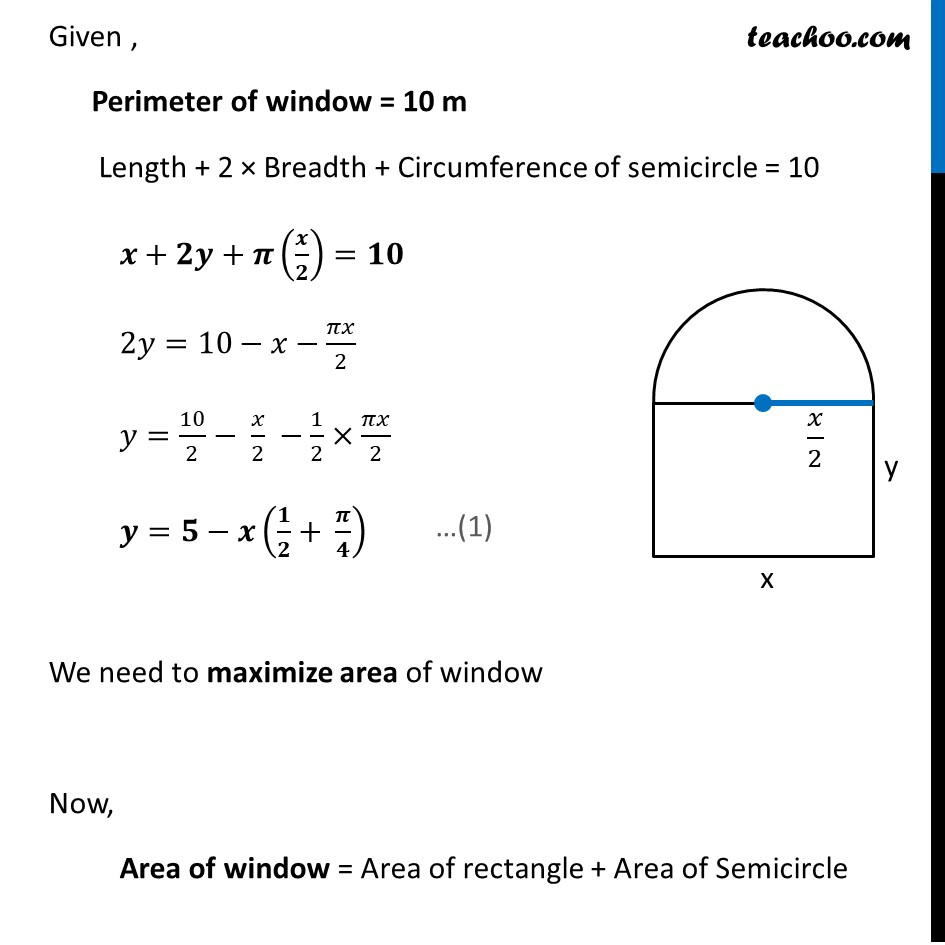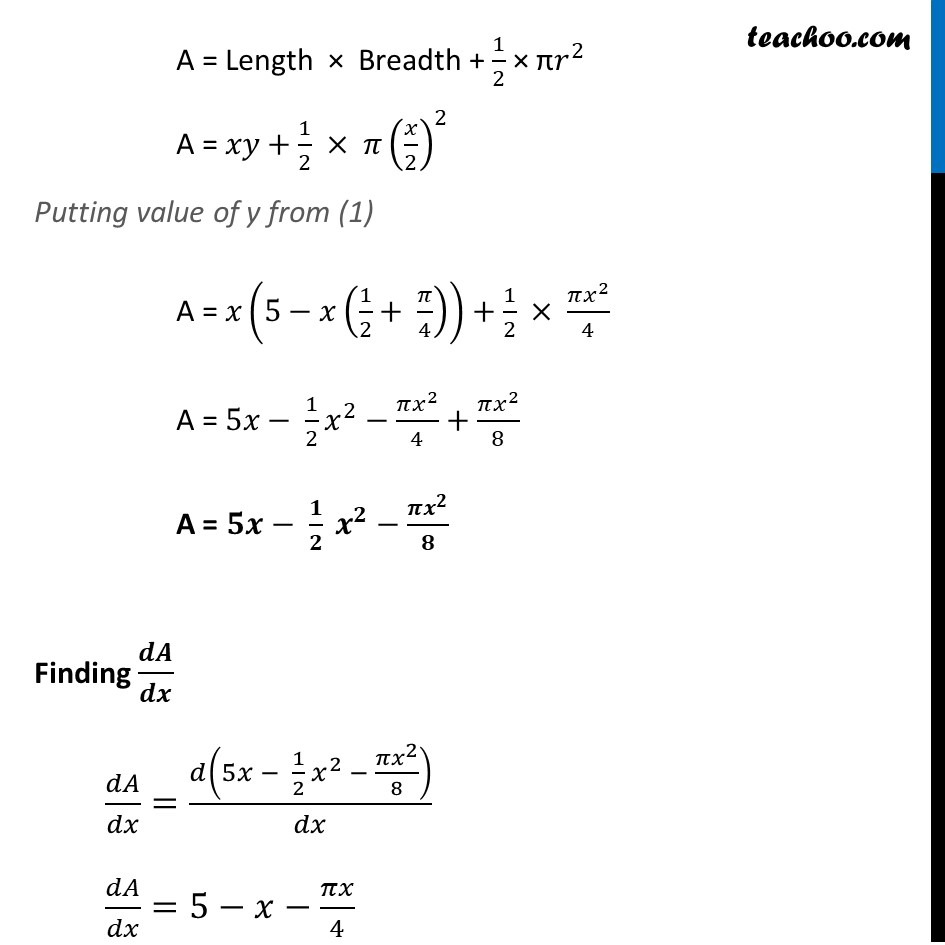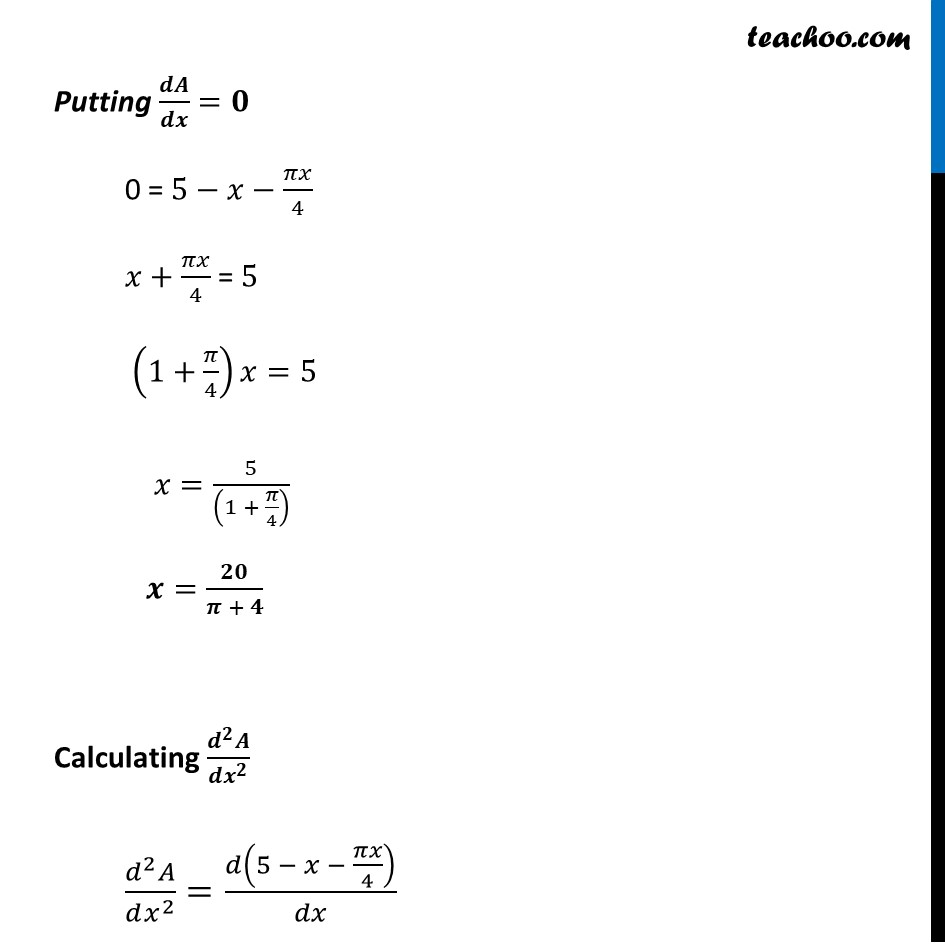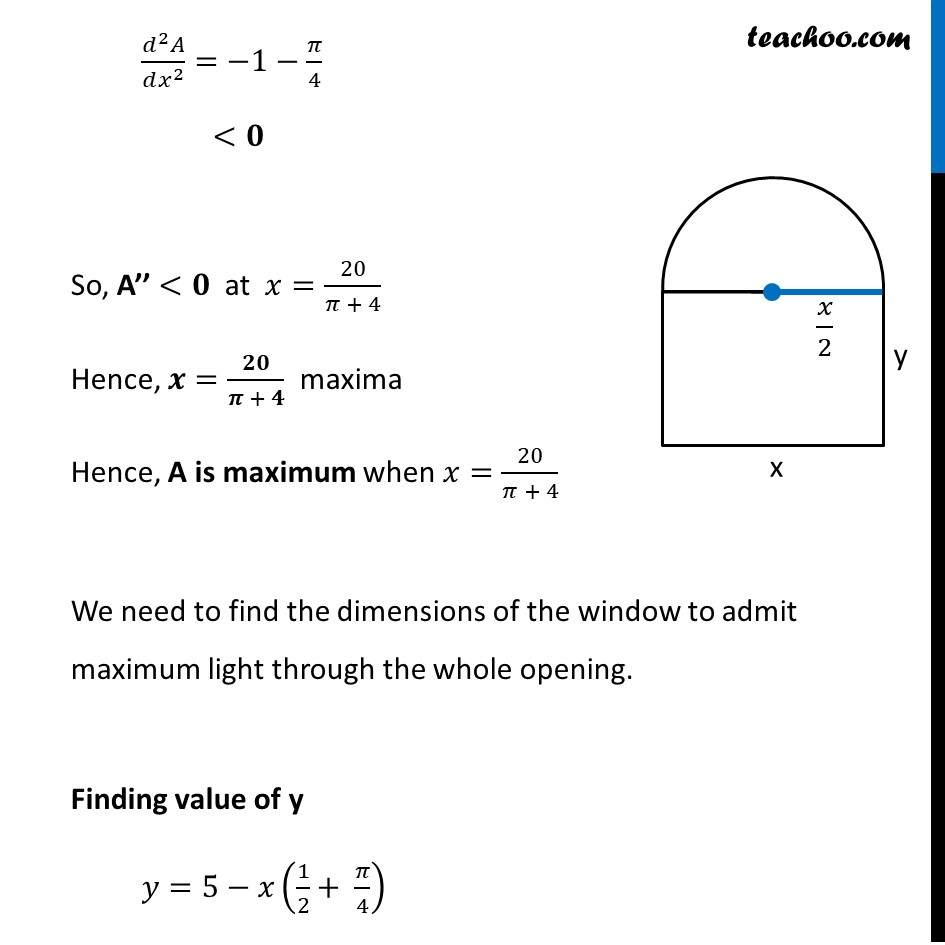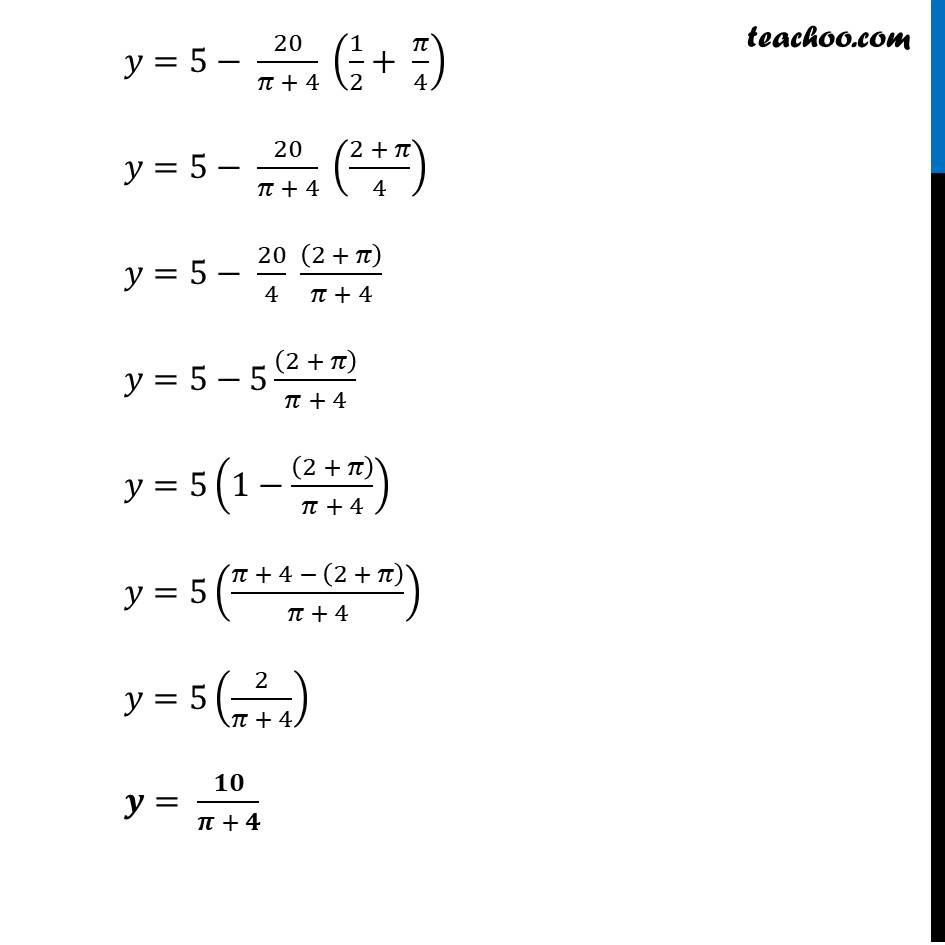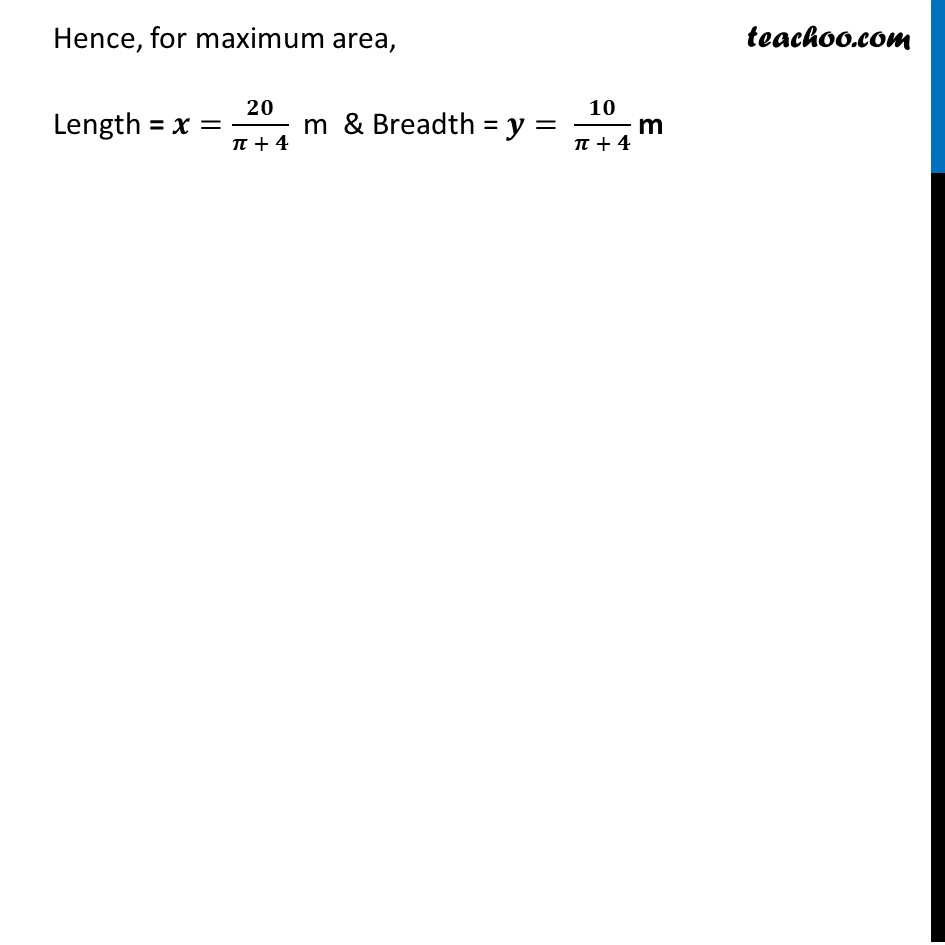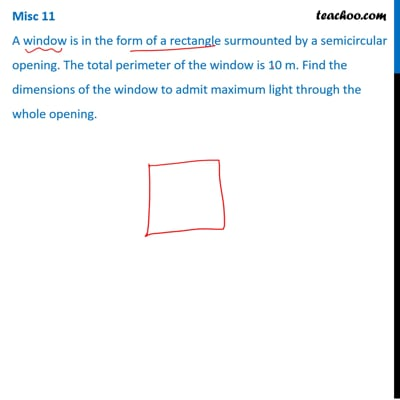This video is only available for Teachoo black users

Introducing your new favourite teacher - Teachoo Black, at only ₹83 per month

### Transcript

Misc 11 A window is in the form of a rectangle surmounted by a semicircular opening. The total perimeter of the window is 10 m. Find the dimensions of the window to admit maximum light through the whole opening.Let Length of rectangle be x & Breadth of rectangle be y Here Diameter of semicircle = x ∴ Radius of semicircle = 𝒙/𝟐 Given , Perimeter of window = 10 m Length + 2 × Breadth + Circumference of semicircle = 10 𝒙+𝟐𝒚+𝝅(𝒙/𝟐)=𝟏𝟎 2𝑦=10−𝑥−𝜋𝑥/2 𝑦=10/2− 𝑥/2 −1/2×𝜋𝑥/2 𝒚=𝟓−𝒙(𝟏/𝟐+ 𝝅/𝟒) We need to maximize area of window Now, Area of window = Area of rectangle + Area of Semicircle A = Length × Breadth + 1/2 × π𝑟^2 A = 𝑥𝑦+1/2 × 𝜋(𝑥/2)^2 Putting value of y from (1) A = 𝑥(5−𝑥(1/2+ 𝜋/4))+1/2 × (𝜋𝑥^2)/4 A = 5𝑥− 1/2 𝑥^2−(𝜋𝑥^2)/4+(𝜋𝑥^2)/8 A = 𝟓𝒙− 𝟏/𝟐 𝒙^𝟐−(𝝅𝒙^𝟐)/𝟖 Finding 𝒅𝑨/𝒅𝒙 𝑑𝐴/𝑑𝑥=𝑑(5𝑥 − 1/2 𝑥^2 − (𝜋𝑥^2)/8)/𝑑𝑥 𝑑𝐴/𝑑𝑥=5−𝑥−𝜋𝑥/4 Putting 𝒅𝑨/𝒅𝒙=𝟎 0 = 5−𝑥−𝜋𝑥/4 𝑥+𝜋𝑥/4 = 5 (1+𝜋/4)𝑥=5 𝑥=5/((1 + 𝜋/4) ) 𝒙=𝟐𝟎/(𝝅 + 𝟒) Calculating (𝒅^𝟐 𝑨)/(𝒅𝒙^𝟐 ) (𝑑^2 𝐴)/(𝑑𝑥^2 )=𝑑(5 − 𝑥 − 𝜋𝑥/4)/𝑑𝑥 (𝑑^2 𝐴)/(𝑑𝑥^2 )=−1−𝜋/4 <𝟎 So, A’’ <𝟎 at 𝑥=20/(𝜋 + 4) Hence, 𝒙=𝟐𝟎/(𝝅 + 𝟒) maxima Hence, A is maximum when 𝑥=20/(𝜋 + 4) We need to find the dimensions of the window to admit maximum light through the whole opening. Finding value of y 𝑦=5−𝑥(1/2+ 𝜋/4) 𝑦=5− 20/(𝜋 + 4) (1/2+ 𝜋/4) 𝑦=5− 20/(𝜋 + 4) ((2 + 𝜋)/4) 𝑦=5− 20/4 ((2 + 𝜋))/(𝜋 + 4) 𝑦=5−5 ((2 + 𝜋))/(𝜋 + 4) 𝑦=5(1−((2 + 𝜋))/(𝜋 + 4)) 𝑦=5((𝜋 + 4 − (2 + 𝜋))/(𝜋 + 4)) 𝑦=5(2/(𝜋 + 4)) 𝒚= 𝟏𝟎/(𝝅 + 𝟒) Hence, for maximum area, Length = 𝒙=𝟐𝟎/(𝝅 + 𝟒) m & Breadth = 𝒚= 𝟏𝟎/(𝝅 + 𝟒) m